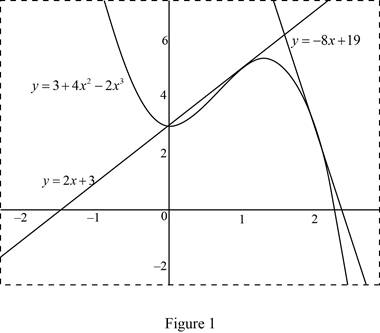# The slope of the tangent line to the curve.### Single Variable Calculus: Concepts...

4th Edition
James Stewart
Publisher: Cengage Learning
ISBN: 9781337687805### Single Variable Calculus: Concepts...

4th Edition
James Stewart
Publisher: Cengage Learning
ISBN: 9781337687805

#### Solutions

Chapter 2.6, Problem 9E

(a)

To determine

## To find: The slope of the tangent line to the curve.

Expert Solution

The slope of the tangent line to the curve y=3+4x22x3 at the point where

x=a is m=8a6a2.

### Explanation of Solution

Given:

The equation of the curve is y=3+4x22x3.

The curve passing through the points (1, 5) and (2, 3).

Formula used:

The slope of the tangent curve y=f(x) at the point P(a,f(a)) is,

m=limh0f(a+h)f(a)h (1)

Calculation:

Obtain the slope of the tangent to the curve at the point x=a.

Since f(x)=3+4x22x3, substitute f(a)=3+4a22a3 and f(a+h)=3+4(a+h)22(a+h)3 in equation (1),

m=limh0f(a+h)f(a)h=limh0(3+4(a+h)22(a+h)3)(3+4a22a3)h=limh0(3+4(a2+h2+2ah)2(a3+3a2h+3ah2+h3))(3+4a22a3)h=limh0(3+4a2+4h2+42ah2a323a2h23ah22h3)(3+4a22a3)h

Perform the mathematical operations as shown below.

m=limh03+4a2+4h2+8ah2a36a2h6ah22h334a2+2a3h=limh0(33)+(4a24a2)+(2a32a3)+4h2+8ah6a2h6ah22h3h=limh04h2+8ah6a2h6ah22h3h=limh0h(4h+8a6a26ah2h2)h

Since the limit h approaches 0 but not equal to 1, cancel the common term h(0) from both the numerator and the denominator,

m=limh0(4h+8a6a26ah2h2)=(4(0)+8a6a26a(0)2(0)2)=0+8a6a200=8a6a2

Thus, the slope of the tangent line to the curve at the point x=a is m=8a6a2.

(b)

To determine

### To find: The equation of the tangent lines to the curve y=3+4x2−2x3 at the points (1,5) and (2, 3).

Expert Solution

The equation of the tangent lines to the curve y=3+4x22x3 at the points (1, 5) and

(2, 3) are y=2x+3 and y=8x+19 respectively.

### Explanation of Solution

Formula used:

The equation of the tangent line to the curve y=f(x) at the point (a,f(a)) is,

yf(a)=f(a)(xa) (2)

Since the tangent line to the curve y=f(x) at (a,f(a)) is the line through the point (a,f(a)) whose slope is equal to the derivative of a, the value of f(a)=8a6a2.

At the point (1, 5), take a=1 and f(a)=5

f(1)=8(1)6(1)2=86=2

Substitute a=1, f(a)=5 and f(a)=2 in equation (2),

(yf(a))=f(a)(xa)y5=2(x1)y5=2x2

Isolate y as shown below:

y=2x2+5=2x+3

Thus, the equation of the tangent line is y=2x+3.

Obtain the equation of the tangent line at the point (2, 3).

Since the tangent line to the curve y=f(x) at (a,f(a)) is the line through the point (a,f(a)) whose slope is equal to the derivative of a, the value of f(a)=8a6a2.

At the point (2, 3), take a=2 and f(a)=3

f(2)=8(2)6(2)2=1624=8

Substitute a=2, f(a)=3 and f(a)=8 in equation (2).

(yf(a))=f(a)(xa)y3=8(x2)y3=8x+16

Isolate y as shown below:

y=8x+16+3=8x+19

Thus, the equation of the tangent line is y=8x+19.

(c)

To determine

Expert Solution

### Explanation of Solution

Given:

The equation of the curve is y=3+4x22x3.

The equation of the tangent lines are y=2x+3 and y=8x+19.

Graph:

Use the online graphing calculator to draw the graph of the functions as shown below in Figure 1.Notice that, the two lines y=2x+3 and y=8x+19 touches on the curve y=3+4x22x3 at a unique point. That is, the two lines are tangent to the curve y=3+4x22x3.

### Have a homework question?

Subscribe to bartleby learn! Ask subject matter experts 30 homework questions each month. Plus, you’ll have access to millions of step-by-step textbook answers!